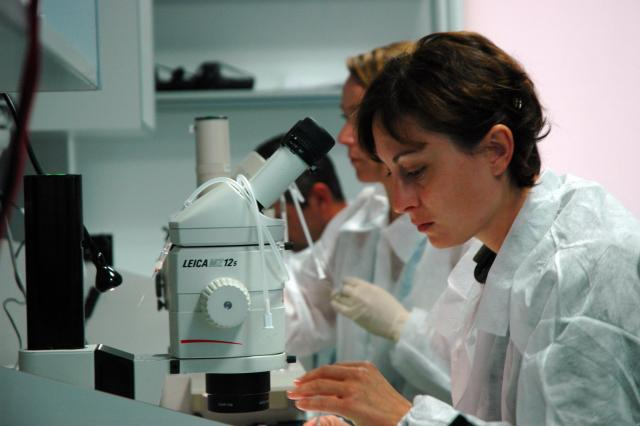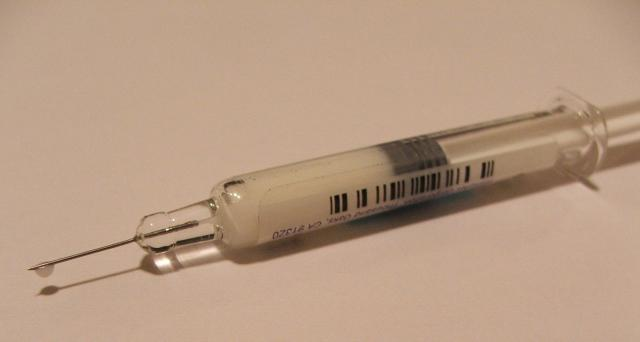Topics: Hypothesis Testing

The P value is used all over statistics, from t-tests to regression analysis. Everyone knows that you use P values to determine statistical significance in a hypothesis test. In fact, P values often determine what studies get published and what projects get funding.

Despite being so important, the P value is a slippery concept that people often interpret incorrectly. How do you interpret P values?

In this post, I'll help you to understand P values in a more intuitive way and to avoid a very common misinterpretation that can cost you money and credibility.

## What Is the Null Hypothesis in Hypothesis Testing?In order to understand P values, you must first understand the null hypothesis.

In every experiment, there is an effect or difference between groups that the researchers are testing. It could be the effectiveness of a new drug, building material, or other intervention that has benefits. Unfortunately for the researchers, there is always the possibility that there is no effect, that is, that there is no difference between the groups. This lack of a difference is called the null hypothesis, which is essentially the position a devil’s advocate would take when evaluating the results of an experiment.

To see why, let’s imagine an experiment for a drug that we know is totally ineffective. The null hypothesis is true: there is no difference between the experimental groups at the population level.

Despite the null being true, it’s entirely possible that there will be an effect in the sample data due to random sampling error. In fact, it is extremely unlikely that the sample groups will ever exactly equal the null hypothesis value. Consequently, the devil’s advocate position is that the observed difference in the sample does not reflect a true difference between populations.

## What Are P Values?P values evaluate how well the sample data support the devil’s advocate argument that the null hypothesis is true. It measures how compatible your data are with the null hypothesis. How likely is the effect observed in your sample data if the null hypothesis is true?

• High P values: your data are likely with a true null.
• Low P values: your data are unlikely with a true null.

A low P value suggests that your sample provides enough evidence that you can reject the null hypothesis for the entire population.

## How Do You Interpret P Values?In technical terms, a P value is the probability of obtaining an effect at least as extreme as the one in your sample data, assuming the truth of the null hypothesis.

For example, suppose that a vaccine study produced a P value of 0.04. This P value indicates that if the vaccine had no effect, you’d obtain the observed difference or more in 4% of studies due to random sampling error.

P values address only one question: how likely are your data, assuming a true null hypothesis? It does not measure support for the alternative hypothesis. This limitation leads us into the next section to cover a very common misinterpretation of P values.

## P Values Are NOT the Probability of Making a Mistake

Incorrect interpretations of P values are very common. The most common mistake is to interpret a P value as the probability of making a mistake by rejecting a true null hypothesis (a Type I error).

There are several reasons why P values can’t be the error rate.

First, P values are calculated based on the assumptions that the null is true for the population and that the difference in the sample is caused entirely by random chance. Consequently, P values can’t tell you the probability that the null is true or false because it is 100% true from the perspective of the calculations.

Second, while a low P value indicates that your data are unlikely assuming a true null, it can’t evaluate which of two competing cases is more likely:

• The null is true but your sample was unusual.
• The null is false.

Determining which case is more likely requires subject area knowledge and replicate studies.

Let’s go back to the vaccine study and compare the correct and incorrect way to interpret the P value of 0.04:

• Correct: Assuming that the vaccine had no effect, you’d obtain the observed difference or more in 4% of studies due to random sampling error.

• Incorrect: If you reject the null hypothesis, there’s a 4% chance that you’re making a mistake.

To see a graphical representation of how hypothesis tests work, see my post: Understanding Hypothesis Tests: Significance Levels and P Values.

## What Is the True Error Rate?Think that this interpretation difference is simply a matter of semantics, and only important to picky statisticians? Think again. It’s important to you.

If a P value is not the error rate, what the heck is the error rate? (Can you guess which way this is heading now?)

Sellke et al.* have estimated the error rates associated with different P values. While the precise error rate depends on various assumptions (which I discuss here), the table summarizes them for middle-of-the-road assumptions.

 P value Probability of incorrectly rejecting a true null hypothesis 0.05 At least 23% (and typically close to 50%) 0.01 At least 7% (and typically close to 15%)

Do the higher error rates in this table surprise you? Unfortunately, the common misinterpretation of P values as the error rate creates the illusion of substantially more evidence against the null hypothesis than is justified. As you can see, if you base a decision on a single study with a P value near 0.05, the difference observed in the sample may not exist at the population level. That can be costly!

Now that you know how to interpret P values, read my five guidelines for how to use P values and avoid mistakes.

You can also read my rebuttal to an academic journal that actually banned P values!

An exciting study about the reproducibility of experimental results was published in August 2015. This study highlights the importance of understanding the true error rate. For more information, read my blog post: P Values and the Replication of Experiments.

The American Statistical Association speaks out on how to use p-values!

*Thomas SELLKE, M. J. BAYARRI, and James O. BERGER, Calibration of p Values for Testing Precise Null Hypotheses, The American Statistician, February 2001, Vol. 55, No. 1